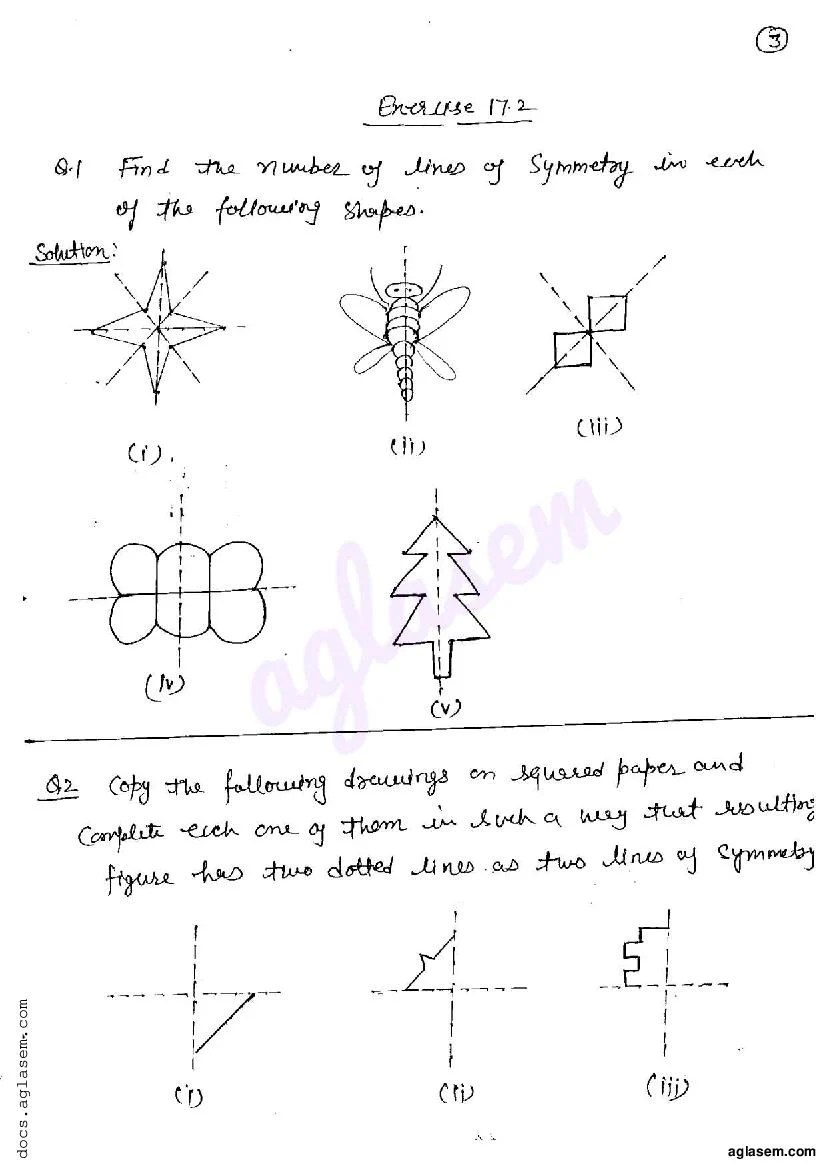# RD Sharma Solutions Class 6 Chapter 17 Symmetry Exercise 17.2

Here you can get free RD Sharma Solutions for Class 6 Maths Chapter 17 Symmetry Exercise 17.2. All RD Sharma Book Solutions are given here exercise wise for the chapter Symmetry. RD Sharma Solutions are helpful in the preparation of several school level, graduate and undergraduate level competitive exams. Practicing questions from RD Sharma Mathematics Solutions for Class 6 Chapter 17 Symmetry is proven to enhance your math skills.

 Class: Class 6th Chapter: Chapter 17 Exercise: Exercise 17.2 Name: Symmetry

## RD Sharma Solutions Class 6 Chapter 17 Symmetry Exercise 17.2

RD Sharma Class 6 Solutions Chapter 17 for Symmetry Exercise 17.2 are given below.

RD Sharma Solutions Class 6 Maths Chapter 17 Symmetry Exercise 17.2